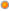## PMAT 22213: Mathematical Methods for Computing

User Rating: 5 / 5Course Code            : PMAT 22213

Title                          : Mathematical Methods for Computing

Learning Outcomes:

At the end of this course, the student should be able

• solve linear ordinary differential equations
• acquaint with the basic mathematical tools Laplace transform, Fourier series, and Fourier Transforms

Course Contents:

Ordinary differential equations: Second & higher order linear differential equations with constant coefficient, General solution of homogenous and non-homogeneous equations, method of variation of parameters, Euler-Cauchy equation, simultaneous liner equations.

Special functions: Power series method, Forbenious method, Legendre equation, Legendre polynomials, Bessel equations, Bessel function of first kind, Orthogonal property, Rodrigues’ formula.

Laplace Transforms: Basic properties, Laplace transform of derivatives and integrals, Inverse Laplace transform, differentiation and integration of Laplace transforms, convolution theorem, unit of step function, Periodic function, Laplace transform to IVP and boundary value problem application, system of linear simultaneous differential equations.

Fourier series: Fourier series, Dirichlet conditions, Even and odd functions, half range series, harmonic analysis.

Method of Teaching and Learning: A combination of lectures and tutorial discussions.

Assessment: Based on tutorials, tests and end of course examination.